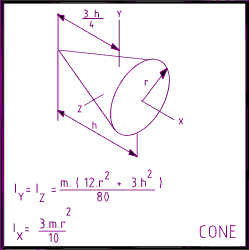Mass Moment of Inertia of a Solid Rod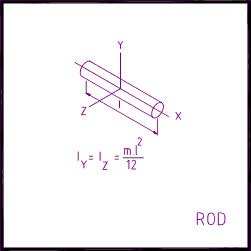Mass Moment of Inertia of a Solid Cylinder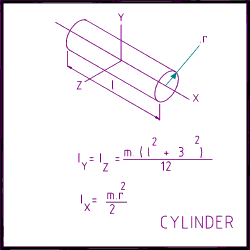Mass Moment of Inertia of a Rectangular Prism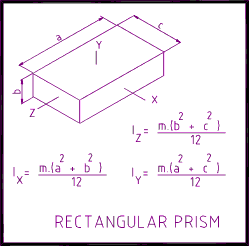Mass Moment of Inertia of a Hollow Cylinder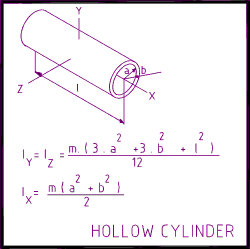Mass Moment of Inertia of a Solid Sphere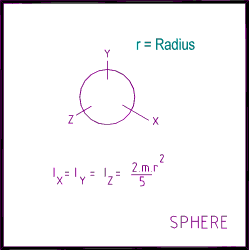Mass Moment of Inertia of a Solid Cone